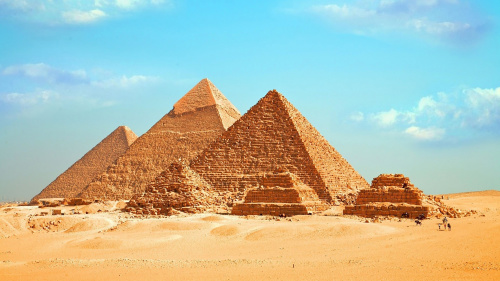# 金字塔神奇数字“142857”蕴藏宇宙秘密？(图)

2019-10-20 07:00 桌面版 正體 0“142857”这一组阿拉伯数字，看起来似乎没什么特别的地方，但往下看你就会发现它的神奇，因为它是一个“走马灯数”（一个n位数乘以1至n的总数是原数的循环排列）。

142857×1=142857

142857×2=285714

142857×3=428571

142857×4=571428

142857×5=714285

142857×6=857142

142+857=999

14+28+57=99

20408+122449=142857

142857×1=142857（原数字）

142857×2=285714（轮值）

142857×3=428571（轮值）

142857×4=571428（轮值）

142857×5=714285（轮值）

142857×6=857142（轮值）

142857×7=999999（放假由9代班）

142857×8=1142856（7分身，变成头尾的1和6，数列内少了7）

142857×9=1285713（4分身，变成头尾的1和3，数列内少了4）

142857×10=1428570（1分身）

142857×11=1571427（8分身）

142857×12=1714284（5分身）

142857×13=1857141（2分身）

142857×14=1999998（9分身）

1+4+2+8+5+7=27=2+7=9

1+9+9+9+9+9+8=54=5+4=9